Trees

# Trees

2020-02-17T23:46:37.025Z

A tree has nodes (containers of values) and edges (connecting lines). Relationships are parent-child. Bottom nodes (childless nodes) are leaves. Each parent has only one other node referencing it.

The top node is the root. The root is the only node from which you have a path to every other node. The root node is inherently the start of any tree.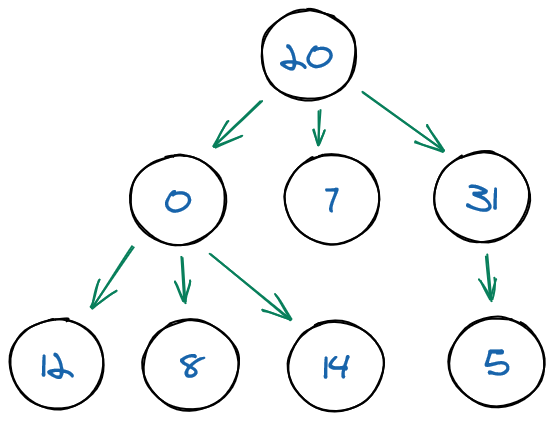Tree

Trees are often used for representing hierarchical data: databases, autocompletion, compilers, etc.

• Node depth is the number of edges from the root to that node.
• Node height is the number of edges from a leaf to that node. Root height is thus also called tree height.

Formula for calculating node height: `1 + max(height(leftSubtree), height(rightSubtree))`

Three important kinds of trees are:

• binary trees
• binary search trees
• heaps

In a binary tree, each parent has zero, one or two nodes, i.e. each node has no more than two children, referred to as "left" and "right".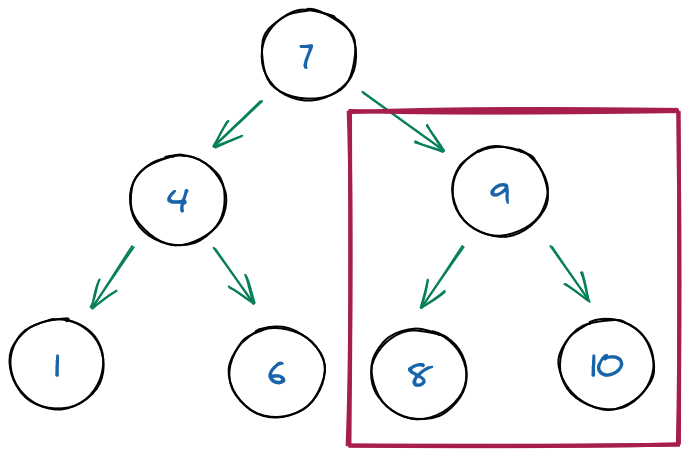Binary tree

In a binary search tree, each parent has zero, one or two nodes, and the value of any node is higher than the value of its left child and lower than the value of its right child. This BST relationship obtains for the whole tree and its subsections, allowing you to find a value without traversing the entire tree. While ordered arrays are just as fast as binary trees when searching data, binary trees are significantly faster when it comes to inserting and deleting data.

In a BST, the value held by a node's left child is less than or equal to its own value, and the value held by a node's right child is greater than or equal to its value. In effect, the data in a BST is sorted by value: all the descendants to the left of a node are less than or equal to the node, and all the descendants to the right of the node are greater than or equal to the node. BSTs are so common that many people mean a BST when they say "tree."

Lookup is fast because on each iteration you eliminate half the remaining nodes from your search by choosing to follow the left subtree or the right subtree. In the worst case, you will know whether the lookup was successful by the time there is only one node left to search. Therefore, the running time of the lookup is equal to the number of times that you can halve `n` nodes before you get to `1`. Whenever we throw out half of the items in a search, we have logarithmic time complexity. Lookup is an `O(log n)` operation in a balanced binary search tree.

Binary search trees have other important properties. For example, you can obtain the smallest element by following all the left children and the largest element by following all the right children.

Operation BST runtime
lookup `O(log n)`
insertion `O(log n)`
deletion `O(log n)`

Binary tree problems can often be solved more quickly than equivalent generic tree problems, but they are no less challenging. Because time is at a premium in an interview, most tree problems will be binary tree problems. If an interviewer says "tree," it's a good idea to clarify whether it refers to a generic tree or a binary tree.

## Implementation

Implementing a BST in Java:

``````public class Tree {
private class Node {
int value;
Node leftChild;
Node rightChild;

public Node(int value) {
this.value = value;
}

@Override
public String toString() {
return "Node=" + value;
}
}

private Node root;

private void insert(int value)  {
if (root == null) {
root = new Node(value);
return;
}

var current = root;
while (true) {
// if value being added is lower than current, go to left subtree
if (value < current.value) {
// if current has no left child, current is parent, so
// insert value being added as its child
if (current.leftChild == null) {
current.leftChild = new Node(value);
break;
}
// if current has a left child,
// then move current to that left child and check again
current = current.leftChild;
} else {
if (current.rightChild == null) {
current.rightChild = new Node(value);
break;
}
current = current.rightChild;
}
}
}

public boolean find(int value) {
var current = root;
while (current != null) {
if (value < current.value) {
current = current.leftChild;
} else (value > current.value) {
current = current.rightChild;
} else {
return true;
}
}
return false;
}
}
``````

Implementing a BST in JavaScript:

``````class Node {
constructor(data) {
this.data = data;
this.left = null;
this.right = null;
}
insert(data) {
if (data < this.data && this.left) {
this.left.insert(data);
} else if (data < this.data) {
this.left = new Node(data);
} else if (data > this.data && this.right) {
this.right.insert(data);
} else if (data > this.data) {
this.right = new Node(data);
}
}
contains(data) {
if (this.data === data) return this;

if (this.data < data && this.right) {
return this.right.contains(data);
} else if (this.data > data && this.left) {
return this.left.contains(data);
}

return null;
}
}
``````

## Tree search and traversal

One way to search a tree is to do a breadth-first search (BFS). In a BFS you start with the root, move left to right across the second level, then move left to right across the third level, and so forth. A BFS uses additional memory because it is necessary to track the child nodes for all nodes on a given level while searching that level.

Another common way to search for a node is by using a depth-first search (DFS). A depth-first search follows one branch of the tree down as many levels as possible until the target node is found or the end is reached. When the search can't go down any farther, it is continued at the nearest ancestor with unexplored children. DFS has lower memory requirements than BFS because it is not necessary to store all the child pointers at each level.

If you have additional information on the likely location of your target node, one or the other of these algorithms may be more efficient. For instance, if your node is likely to be in the upper levels of the tree, BFS is most efficient. If the target node is likely to be in the lower levels of the tree, DFS has the advantage that it doesn't examine any single level last. (BFS always examines the lowest level last.)

A traversal is just like a search, except that instead of stopping when you find a particular target node, you visit every node in the tree. Often this is used to perform some operation on each node in the tree. The process of visiting every node in a data structure is known as "traversing" the data structure.

Two ways to traverse a tree:

Breadth-first traversal, also known as level-order traversal: We visit the nodes at a given level before going down to the nodes in the next level.Depth-first traversal: We visit the child nodes before going to the next parent. It be pre-order, in-order or post-order traversal.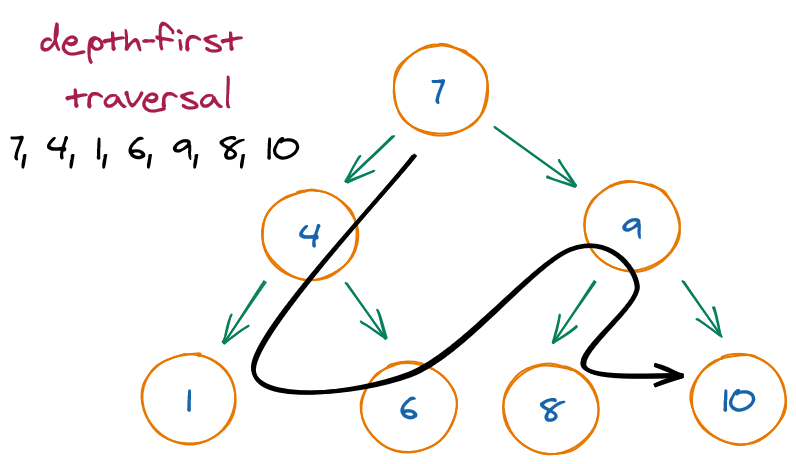Depth-first search

The three most common types of depth-first traversals for binary trees:

• Pre-order: Performs the operation first on the node itself, then on its left descendants, and finally on its right descendants. In other words, a node is always operated on before any of its descendants.
• In-order: Performs the operation first on the node's left descendants, then on the node itself, and finally on its right descendants. In other words, the left subtree is operated on first, then the node itself, and then the node's right subtree.
• Post-order: Performs the operation first on the node's left descendants, then on the node's right descendants, and finally on the node itself. In other words, a node is always operated on after all its descendants.
Order Sequence
Pre-order root, left, right
In-order left, root, right
Post-order left, right, root
::scale-40[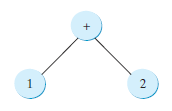]
::figure-caption[Pre-order, in-order and post-order traversal]

In-order is `1 + 2`, post-order is `1 2 +`, and pre-order is `+ 1 2`.

Recursion is usually the simplest way to implement a depth-first traversal.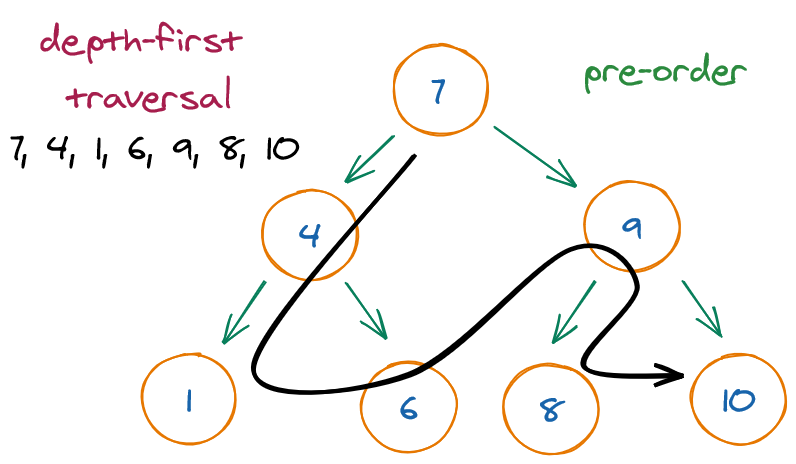Pre-order traversal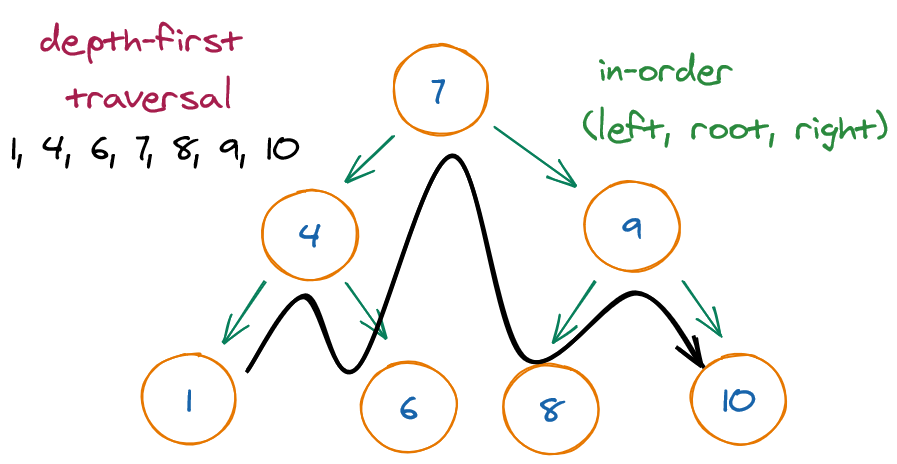In-order traversal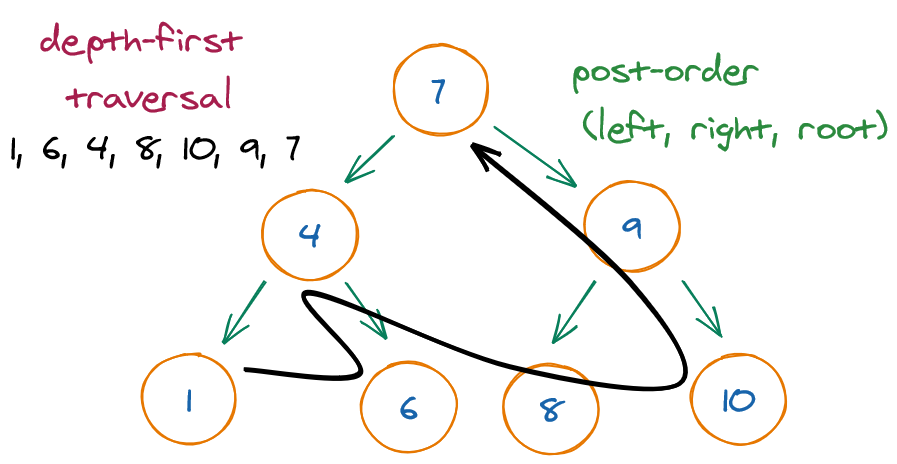Post-order traversal

Tree traversal can be done with or without recursion.

Without recursion:

``````// 4! = 4 x 3 x 2 x 1
public int factorial (int n) {
var factorial = 1;
for (var i = n; i > 1; i--)
factorial *= i;
return factorial;
}
``````

With recursion:

``````// 4! = 4 x 3!
// n! = n x (n - 1)!
public int factorial (int n) {
// base condition
if (n == 0)
return 1;

// recursive section
return n * factorial(n - 1);
}
``````

### Traversal implementation

The following traversal implementations continue the `Tree` implemented above.

Depth-first, pre-order traversal:

``````public void traversePreOrder(Node root) {
if (root == null)
return;
System.out.println(root.value);
traversePreOrder(root.leftChild);
traversePreOrder(root.rightChild);
}
``````

Depth-first, in-order traversal:

``````// highlight-range{4-5}
public void traverseInOrder(Node root) {
if (root == null)
return;
traverseInOrder(root.leftChild); // ← line swapped
System.out.println(root.value); // ← line swapped
traverseInOrder(root.rightChild);
}
``````

Depth-first, post-order traversal:

``````public void traversePostOrder(Node root) {
if (root == null)
return;
traversePostOrder(root.leftChild); // ← line swapped
traversePostOrder(root.rightChild); // ← line swapped
System.out.println(root.value); // ← line swapped
}
``````

``````class Node {
constructor(data) {
this.data = data;
this.children = [];
}
this.children.push(new Node(data));
}
remove(data) {
this.children = this.children.filter(node => node.data !== data);
}
}

class Tree {
constructor() {
this.root = null;
}
traverseBFS(fn) {
const arr = [this.root];
while (arr.length) {

// take out first item (root)
const node =  arr.shift();

// populate arr with root's children
// adding them to the END OF THE ARRAY (push → breadth-first)
arr.push(...node.children);
fn(node);
}
}
traverseDFS(fn) {
const arr = [this.root];
while (arr.length) {

// take out first item (root)
const node =  arr.shift();

// populate arr with root's children
// adding them to the START OF THE ARRAY (unshift → depth-first)
arr.unshift(...node.children);
fn(node);
}
}
}
``````

## Common operations

Calculating the height of a subtree:

``````public int getHeight(Node root) {

if (root == null)
return -1;

// base condition: if leaf node
if (root.leftChild == null && root.rightChild == null) {
return 0;
}

return 1 + Math.max(height(root.leftChild), height(root.rightChild));
}
``````

Finding the minimum value in a binary tree:

``````public int min(Node root) {
// base condition: if leaf node
if (root.leftChild == null && root.rightChild == null) {
return root.value;
}

var left = min(root.leftChild);
var right = min(root.rightChild);
return Math.min(Math.min(left, right), root.value);
}
``````

Determining if two trees are equal:

``````public boolean areEqual(rootFirst, rootSecond) {
if (first == null && second == null)
return true;

if (first != null && second != null)
return first.value == second.value
&& isEqual(rootFirst.leftChild, rootSecond.leftChild);
&& isEqual(rootFirst.rightChild, rootSecond.rightChild);

return false;
}
``````

Determining if binary tree is binary search tree:

``````public boolean isBinarySearchTree(Node root, int min, int max) {
if (root == null)
return true;

if (root.value < min || root.value < max)
return false;

isBinarySearchTree(root.leftChild, min, root.value - 1) &&
isBinarySearchTree(root.rightChild, root.value + 1, max);
}
``````

Printing nodes at k distance from root:

``````public void printNodesAtDistance(Node root, int distance) {
if (root == null)
return;

if (distance == 0) {
System.out.println(root.value);
return;
}

printNodesAtDistance(root.leftChild, distance - 1);
printNodesAtDistance(root.rightChild, distance - 1);
}
``````

Getting nodes at k distance from root:

``````public void getNodesAtDistance(Node root, int distance, ArrayList<Integer> list) {
if (root == null)
return;

if (distance == 0) {
return;
}

getNodesAtDistance(root.leftChild, distance - 1);
getNodesAtDistance(root.rightChild, distance - 1);
return list;
}
``````

Traversing a tree breadth-first (level-order):

``````public void traverseLevelOrder() {
for (var i = 0; i <= height(), i++) {
for (var value : getNodesAtDistance(i)) {
System.out.println(value);
}
}
}
``````
``````class Solution {
public Node bstFromPreorder(int[] preorder) {
return helper(preorder, 0, preorder.length - 1);
}

private Node helper(int[] preorder, int start, int end) {
if (start > end) return null;

// get first node in array, i.e. root
Node node = new Node(preorder[start]);
int i;

// loop over array
for (i = start; i <= end; i++) {
// if array element is larger than root, terminate loop
if (preorder[i] > node.val)
break;
}

node.left = helper(preorder, start + 1, i - 1);
node.right = helper(preorder, i, end);
return node;
}
}
``````